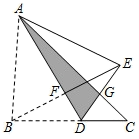.如图, 三角形纸片 $A B C$, 点 $D$ 是 $B C$ 边上一点, 连接 $A D$, 把 $\triangle A B D$ 沿着 $A D$ 翻折, 得到 $V A E D, D E$ 与 $\mathrm{AC}$ 交于点 $G$, 连接 $B E$ 交 $A D$ 于点 $F$. 若 $D G=G E, A F=3, B F=2, \mathrm{~V} A D G$ 的面积为 2 , 则点 $F$ 到 $B C$ 的距离为$\text{A.}$ $\frac{\sqrt{5}}{5}$ $\text{B.}$ $\frac{2 \sqrt{5}}{5}$ $\text{C.}$ $\frac{4 \sqrt{5}}{5}$ $\text{D.}$ $\frac{4 \sqrt{3}}{3}$

B

#### 解析：

【详解】解: $\because D G=G E$,
\begin{aligned} & \therefore S_{\triangle A D G}=S_{\triangle A E G}=2, \\ & \therefore S_{\triangle A D E}=4, \end{aligned}

\begin{aligned} & \therefore S_{\triangle A B D}=S_{\triangle A D E}=4, \angle B F D=90^{\circ}, \\ & \therefore \frac{1}{2} \cdot(A F+D F) \cdot B F=4, \\ & \therefore \frac{1}{2} \cdot(3+D F) \cdot 2=4, \\ & \therefore D F=1, \\ & \therefore D B=\sqrt{B F^2+D F^2}=\sqrt{1^2+2^2}=\sqrt{5}, \end{aligned}

$$\therefore h=\frac{2 \sqrt{5}}{5} \text {, }$$

①点击 首页查看更多试卷和试题 , 点击查看 本题所在试卷